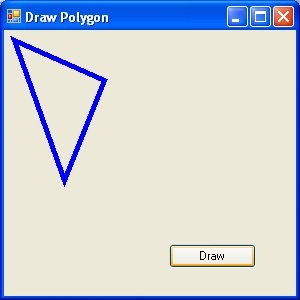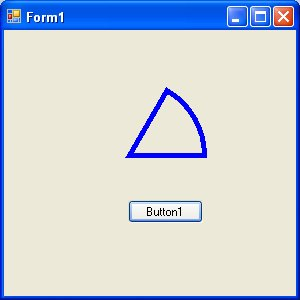# Visual Basic 2013 Lesson 29 : Drawing Polygon and Pie

### 29.1: Drawing a Polygon

A Polygon is a closed plane figure bounded by three or more straight sides. In order to draw a polygon on the screen, you have to define the coordinates of all the points (also known as vertices) that joined up to form the polygon.

The syntax to define the points of a polygon with vertices A1, A2, A3,  A4…….An is as follows;

```Dim A1 As New Point(X1,Y1)Dim A2 As New Point(X2,Y2)
Dim A3 As New Point(X3,Y3)
Dim A4 As New Point(X4,Y4)
.

.

Dim An as New Point(Xn,Yn)
```

After declaring the points, we need to define a point structure that group all the points together using the following syntax: Dim myPoints As Point() = {A1, A2, A3,…., An}.Finally, create the graphics object and use the DrawPolygon method to draw the polygon using the following syntax:

```Dim myGraphics As Graphics = Me.CreateGraphics
myGraphics.DrawPolygon(myPen, myPoints)
```

where myPen is the Pen object created using the following syntax:

```myPen = New Pen(Drawing.Color.Blue, 5)
```

#### 29.1.1 Drawing a Triangle

A triangle is a polygon with three vertices. Here is the code:

```Private Sub BtnDraw_Click(sender As Object, e As EventArgs) Handles BtnDraw.Click
Dim myPen As Pen
Dim A As New Point(10, 10)
Dim B As New Point(100, 50)
Dim C As New Point(60, 150)
Dim myPoints As Point() = {A, B, C}
myPen = New Pen(Drawing.Color.Blue, 5)
Dim myGraphics As Graphics = Me.CreateGraphics
myGraphics.DrawPolygon(myPen, myPoints)

End Sub
```

Running the program produces the image as shown in Figure 29.1#### Figure 29.1

A quadrilateral is a polygon consist of four sides,  so you need to define four vertices. The code is as follows:

```Private Sub BtnDraw_Click(sender As Object, e As EventArgs) Handles BtnDraw.Click
Dim myPen As Pen Dim A As New Point(10, 10)
Dim B As New Point(100, 50)
Dim C As New Point(120, 150)
Dim D As New Point(60, 200)
Dim myPoints As Point() = {A, B, C, D}
myPen = New Pen(Drawing.Color.Blue, 5)
Dim myGraphics As Graphics = Me.CreateGraphics
myGraphics.DrawPolygon(myPen, myPoints)
End Sub
```

The output image is as shown in Figure 29.2### 29.2: Drawing a Pie

In order to draw a pie, you can use the DrawPie method of the graphics object. As usual, you need to create the Graphics and the Pen objects. The syntax for drawing a pie is:

```myGraphics.DrawPie(myPen, X, Y, width,height, StartAngle, SweepAngle)
```

Where X and Y are the coordinates the bounding rectangle, other arguments are self-explanatory. Both StartAngle and SweepAngle are measured in degree. SweepAngle can take possible or negative values. If the value is positive, it sweeps through clockwise direction while negative means it sweep through anticlockwise direction.

#### 29.2.1: Drawing a pie that starts with 0 degrees and sweeps clockwise through 60 degrees.

```Private Sub BtnDraw_Click(sender As Object, e As EventArgs) Handles BtnDraw.Click
Dim myPen As Pen myPen = New Pen(Drawing.Color.Blue, 5)
Dim myGraphics As Graphics = Me.CreateGraphics
myGraphics.DrawPie(myPen, 50,50, 150,150,0,60)
End Sub
```

The output image is as shown in Figure 29.3Figure 29.3